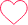# How To Solve Percentage Questions Quickly

## How to solve Percentages Problems Quickly

Here you will find easy tricks on How To Solve Percentage Problems quickly.### Percentage Questions & Definition

• A percentage is a ratio expressed as a fraction whose denominator (bottom) is 100.Thus, x percent means x hundredths, written as x%.
• A percentage is a fraction of an amount expressed as a particular number of hundredths of that amount.

### What is P percent of X?

• Written as an equation: Y = P% × X.
• The ‘what’ is Y that we want to solve for
• Remember to first convert percentage to decimal, dividing by 100
• Solution: Solve for Y using the percentage formula Y = P% × X.

### How to calculate the percentage of a number?

• To calculate the percentage of a number, we need to use a different formula such as:
• P% of Number = X
where X is the required percentage.
• If we remove the % sign, then we need to express the above formulas as;
• P/100 * Number = X

### Type 1: Percentage Problems- based on Mixtures and Allegation

Question 1. A general store shopkeeper sells black pepper at some cost price but he mixes it with papaya’s seed and thereby gains 25%. Find the percentage of papaya’s seed mixed with black pepper?

Options:

A. 25%

B. 30%

C. 20%

D. 10%

Solution:     Let the CP of 1 kg black pepper = Re. 1

The SP of I Kg mixture = Re

1

Gain = 25%

Therefore, CP of 1 Kg

mixture = $\frac{100}{125}$ × 1 = $\frac{4}{5}$

Ratio of black pepper: papaya seed = $\frac{4}{5}$: $\frac{1}{5}$

Hence, percentage of papaya seed in the mixture = $\frac{1}{5}$ × 100 = 20%

Correct option: C

Question 2. A bar tender served a jar full of vodka containing 50% alcohol to a customer. After few minutes, the customer asked the bar tender to replace the vodka by another drink containing 19% alcohol and now the percentage of alcohol was found to be 25%. Find out the quantity of vodka replaced?

Options:

A. $\frac{25}{31}$

B. $\frac{20}{31}$

C. $\frac{1}{2}$

D. $\frac{31}{20}$

Solution:     By the rule of alligation, we have:

Ratio of 1st and 2nd quantities = 6: 25

By the rule of ratio, if x:y is the ratio, to get the quantity of x, the formula is $\frac{x}{x+y}$,and to get the quantity of y, the formula is $\frac{y}{x+y}$

Therefore, required quantity replaced = $\frac{25}{25 + 6}$ = $\frac{25}{31}$

Correct option: A

Question 3. There is one liquid A which contains 25% of water, the liquid B contains 30% of water. A vessel is filled with 6 parts of the liquid A and 4 parts of the liquid B. Find out the percentage of water in the mixture?

Options:

A. 27%

B. 25%

C. 20%

D. 30%

Solution:     Let the capacity of the vessel be 10

So, water from liquid A = 6 × $\frac{25}{100}$ = 1.5

And, water from liquid B = 4 × $\frac{30}{100}$ = 1.2

Total water = 1.5 + 1.2 = 2.7

In terms of percentage = $\frac{2.7}{10}$ ×100 = 27%

Correct option: A

### Type 2: Problems based on Ratios and Fractions

Question 1.If 40% of a number is equal to 2/3rd of another number, what is the ratio of first number to the second number?

Options:

A. 1: 2

B. 2: 3

C. 3: 5

D. 5: 3

Solution:     Let the two numbers be x and y

40% of x = $\frac{2}{3}$y

Then, $\frac{40x}{100}$ = $\frac{2}{3}$y

$\frac{2}{5}$ x = $\frac{2}{3}$y

$\frac{x}{y}$ = $\frac{2}{3}$ ×  $\frac{5}{2}$ = $\frac{5}{3}$

Therefore the ratio = 5: 3

Correct option: D

Question 2: In a box of 8 donuts ,2 donuts have Choco chip sprinkle on them. Find out how many percent of the donuts in the box has Choco chip sprinkle?

Options:

A. 20%

B. 40%

C. 25%

D. 10%

Solution:     $\frac{2}{8}$= $\frac{x}{100}$

8x = 200

x = $\frac{200}{8}$

x = 25%

Correct option: C

Question 3: Two numbers are respectively 40% and 80% more than a third number. The ratio of the two numbers is:

Options:

A. 6: 5

B. 7: 9

C. 2:5

D. 1:2

Solution:     Let the third number x

First number = 40 % more than x = $\frac{140}{100}$ x = $\frac{7}{5}$ x

Second number = 80% more than x = $\frac{180}{100}$ x = $\frac{9}{5}$ x

Therefore their ratio = $\frac{7}{5}$ x : $\frac{9}{5}$ x = 7: 9

Correct option: B

### Related Banners

Get PrepInsta Prime & get Access to all 200+ courses offered by PrepInsta in One Subscription

## Get over 200+ course One Subscription

Courses like AI/ML, Cloud Computing, Ethical Hacking, C, C++, Java, Python, DSA (All Languages), Competitive Coding (All Languages), TCS, Infosys, Wipro, Amazon, DBMS, SQL and others

## Checkout list of all the video courses in PrepInsta Prime Subscription

### 6 comments on “How To Solve Percentage Questions Quickly”

•Hemant

Question 3. Saloni’s salary was decreased by 30% and subsequently increased by 40%. How much percent does she loose?
Options:
A. 16%
B. 15%
C. 12%
D. 13%
Solution: 100*(70/100)*(140/100) = 98
So, 100-98=2
%age = (2/100)*100 = 2%
Is this right?27
•Its wright bro0
•Aparna

in question 2 of type 5 option given is wrong but solution is right0
•HelpPrepInsta

We solve your issue. We hope you are doing great . All the best.

Warm Regards,
Prepinsta Team0
•kaushal0
•0